## Analogy

#### Analogy

Direction: In following questions, select the related figure from the given alternatives.

1. Find the question mark ? figure from answer figure.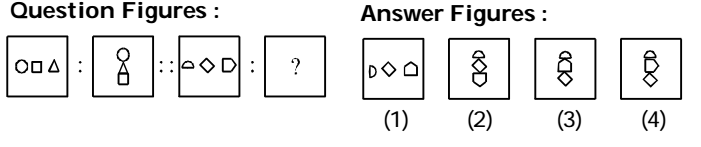1. From first figure to second figure the left most design moves to the top position, the middle design occupies the lowermost position and the right most design comes in the middle.

##### Correct Option: D

From first figure to second figure the left most design moves to the top position, the middle design occupies the lowermost position and the right most design comes in the middle.

1. Find the question mark ? figure from answer figure.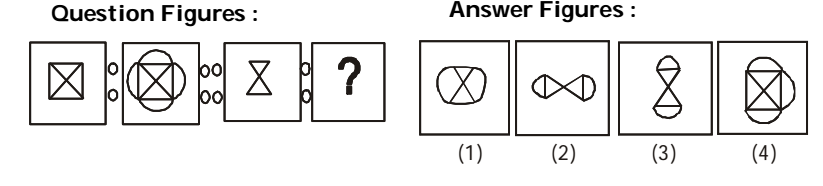1. Semicircles are attached to the parallel sides of the design.

##### Correct Option: C

Semicircles are attached to the parallel sides of the design.

1. Find the question mark ? figure from answer figure.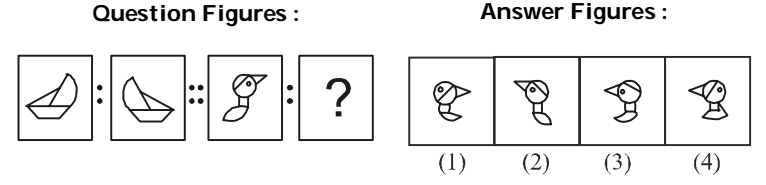1. The second figure is mirror image of the first figure.

##### Correct Option: B

The second figure is mirror image of the first figure.

1. Find the question mark ? figure from answer figure.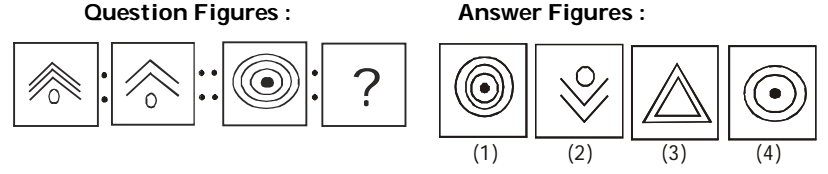1. From first figure to second figure out of three similar designs one is deleted.

##### Correct Option: D

From first figure to second figure out of three similar designs one is deleted.

1. Find the question mark ? figure from answer figure.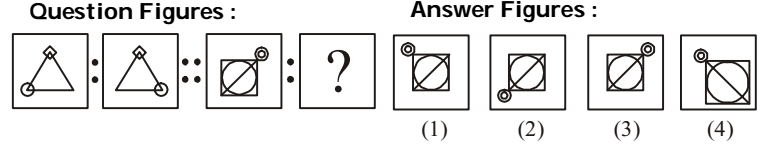1. From first figure to second figure in the first unit of problem figures the circle moves one step in anticlockwise direction.

##### Correct Option: A

From first figure to second figure in the first unit of problem figures the circle moves one step in anticlockwise direction.